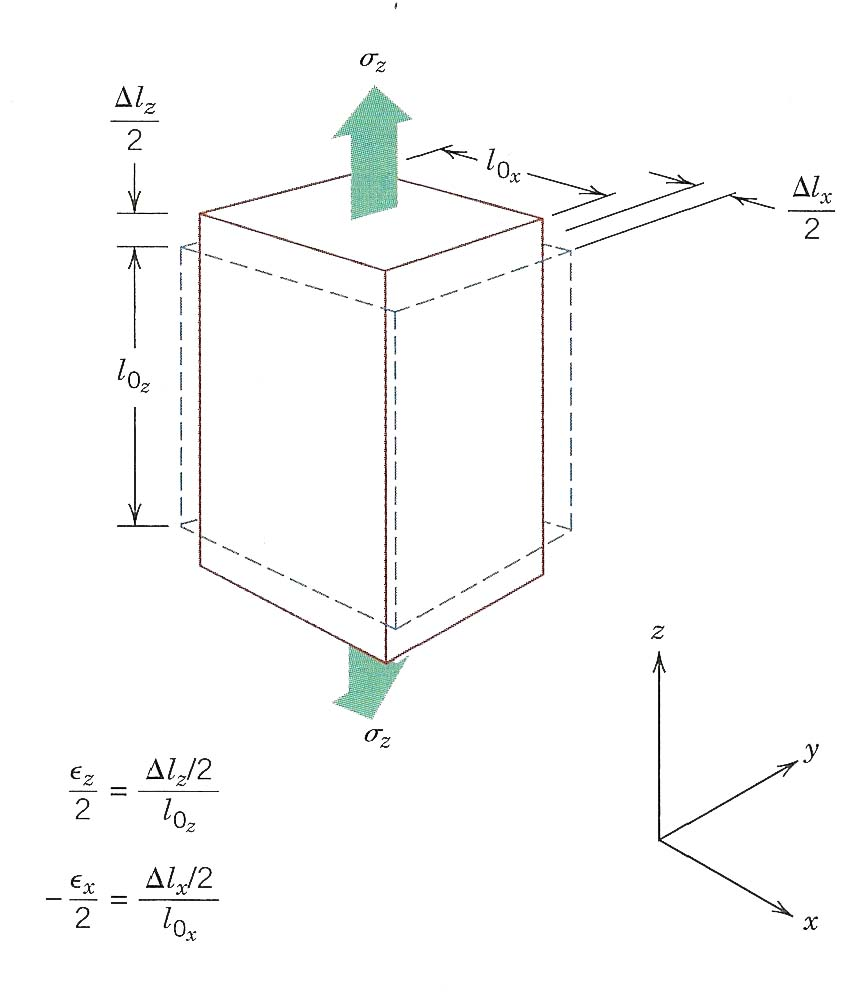# Midterm Review

### Chapter 2

Quantum Number:
Principle Quantum Number n 1,2,3,4,... K,L,M,N,...
Subshells l s, p, d, f
Number of Energy States $$m_{l}$$ 1,3,5,7
Spin $$m_{s}$$ +1/2, -1/2
1. Electron Configuration
1. Ground State
2. Electron configuration of an atom (ground state)
3. Ion electron configuration
1. Pauli Exclusion Principle:
1. Each electron state can hold no more than two electrons, which must have opposite spins.

Bohr model vs. Wave-mechanical model

Electron Location

Valence Electrons

Reactivity of element

$$E = \int (Fdr)$$

Bonding energy: $$E_{0}$$
Equilibrium spacing: $$r_{0}$$

1. Primary Bonding
1.  Ionic Electrons move from element to element Covalent Electrons are shared between elements Metallic Valence forms an electron cloud, shared by all ion cores
2. Secondary bonding
1.  Van der Waals Hydrogen bonding
Bonding Types

% ionic character $$= 1 - exp^{{-(0.25)(X_{A} - X_{B})}^2}x100$$

### Chapter 3

Crystalline vs. crystal structure

Unit Cell

Draw: BCC, FCC, BCC (100), FCC (100), FCC (111)

Atomic Packing Factor: APF = $$\large {{volume\ of\ atoms\ in\ a\ unit\ cell} \over {total\ unit\ cell\ volume}}$$

$$R^3 \over R^3$$

Planar Density: PD = $$\large {{Area\ of\ atoms\ centered\ on\ a\ plane} \over {area\ of\ plane}}$$

$$R^2 \over R^2$$

Linear Density: LD = $$\large {{Length\ occupied\ by\ atoms\ centered\ on\ a\ direction\ vector} \over {length\ of\ direction\ vector}}$$

$$R \over R$$

Theoretical Density

$$\quad \large {\rho = {n*N_{A}\over V_{uc}A}}$$

1. Crystal Systems
1. What are the parameters defining crystal systems?
2. How many crystal systems are there?

Single Crystal vs. Polycrystalline

grain

Grain Boundary

Anisotropic vs. Isotropic

Why might a specimen with grains that are anisotropic, still have isotropic properties?

orientation of grains

### Chapter 4

1. Point Defects
1. Imperfections
1. Vacancies
2. Self-interstitials
2. Impurities
1. Substitution
2. Interstitial
3. $$N_{v} = N exp^{ \large ({-Q_{v}\over kT})}$$
4. Composition (Concentration)
1. Linear Defects
1.  Edge Dislocation b $$\perp$$ to dislocation line Screw Dislocation b // to dislocation line Burgers vector b
1. Interfacial Defects
1. Surface atoms are not bonded to the maximum number of nearest neighbors*, and are therefore in a higher energy state than the atoms at interior positions
2. Grain Boundaries
3. Grain Size
1.  Line intercept method Average Grain Diameter ASTM Method Grain Size Number
*Coordination Number

### Chapter 5

1. Diffusion Types
1. Interdiffusion (impurity diffusion)
2. Self-diffusion
3. Vacancy diffusion
4. Interstitial diffusion

Diffusion flux
$$\quad J = {M \over At}$$

Concentration gradient = $$dC \over dx$$

$${\Delta C \over \Delta x} = {C_{A} - C_{B} \over x_{A} - x_{B}}$$

Fick's first law
$$\quad J = -D {dC \over dx}$$

D: diffusion coefficient

Driving force: that which compels a reaction to occur

1. Diffusion factors
1. Effects on diffusion coefficient, D
1. Vacancy vs. interstitical diffusion coefficients
3. Sufficient Energy
1. $$D=D_{0}exp^{\large ({-Q_{d} \over RT})}$$

### Chapter 6

Three principle ways in which a load may be applied:

tension, compression, shear
Engineering Stress/Strain True Stress/Strain
$$\sigma = {F \over A_{0}}$$ $$\sigma _{T} = {F \over A_{i}}$$
$$\epsilon = {l_{i} - l_{0} \over l_{0}} = {\Delta l \over l_{0}}$$ $$\epsilon_{T} = ln({l_{i} \over l_{0}})$$
##### Hooke's Law

$$\sigma = E \epsilon$$

Only valid for elastic deformation
Mechanical Design Properties
Stiffness
Strength
Ductility
Toughness
Hardness
##### Poisson's Ratio

$$\nu = - \large {{\epsilon _{x} \over \epsilon_{z}} = - {\epsilon_{y} \over \epsilon_{z}}}$$Proportional Limit

Yield Strength

Tensile Strength

%EL = $$\Large {(}$$$$\large{l_{f} - l_{0} \over l_{0}})$$ x 100

%RA = $$\large {({A_{0} - A_{f} \over A_{0}})}$$ x 100